# Thermal Expansion of a Composite PlateThis test lets you check the thermal expansion of a composite plate.

For this test, the reference is the mesh formed of linear hexahedron elements (HE8).
You will use 2D meshes.Reference:

3D model with linear hexahedron elements (HE8), computed with CATIA.

##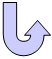Specifications

### Geometry Specifications

 Length: L = 10 000 mm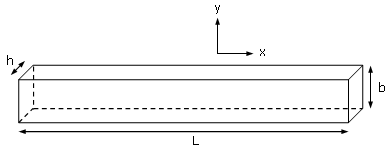Width: b = 1 000 mm Thickness: h = 400 mm divided in two layers

### Analysis Specifications

 First Layer: Young Modulus (material): E = 200 GPa Poisson's Ratio: ν = 0.266 Thermal Expansion Coefficient: α= 1.17 x 10-5/° 3D model (reference):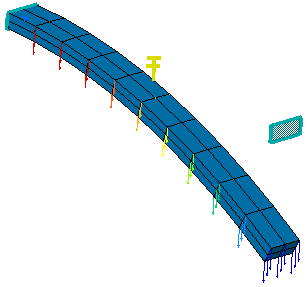Second Layer: Young Modulus (material): E = 200 GPa Poisson's Ratio: ν = 0.266 Thermal Expansion Coefficient: α= 1.2 x 10-2/° Property: Imported Composite Property with Analysis by ply option Mesh Specifications: 10 x 2 elements for linear quadrangle (QD4) and parabolic quadrangle (QD8) elements 10 x 2 x 1 elements for linear hexahedron elements (HE8) 2D model: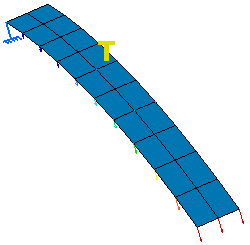Restraints: Clamp on one extremity Loads: temperature field T = 325 Kdeg The environment temperature is T0 = 293.16 Kdeg

##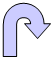Results

The static analysis results correspond to the maximal displacement along the Z component (Tz).

 Quantity Reference Linear Hexahedron (HE8) Computed Results Linear Quadrangle (QD4) Parabolic Quadrangle (QD8)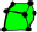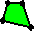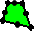Tz max [mm] 73306.6 73193.98 72677.97 Normalized results 1 0.9984 0.9914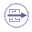To Perform the Test:

The CompositePlateThermoQD4.CATAnalysis document presents a complete analysis of this case, computed with a mesh formed of linear quadrangle elements (QD4).

The CompositePlateThermoHE8.CATAnalysis document presents a complete analysis of this case, computed with a mesh formed of linear hexahedron elements (HE8).

To compute the mesh with parabolic quadrangle elements (QD8), proceed as follow:

1. Open the CompositePlateThermoQD4.CATAnalysis document.

2. Enter the Advanced Meshing Tools workbench.

3. In the specification tree, double-click the mesh part. The Global Parameters dialog box appears.

4. Select Parabolic as element type.

5. Compute the case in the Generative Structural Analysis workbench.